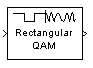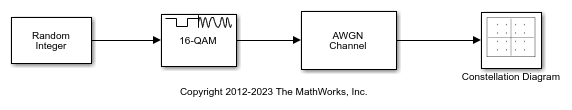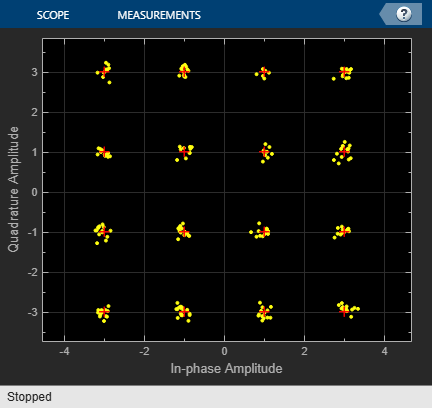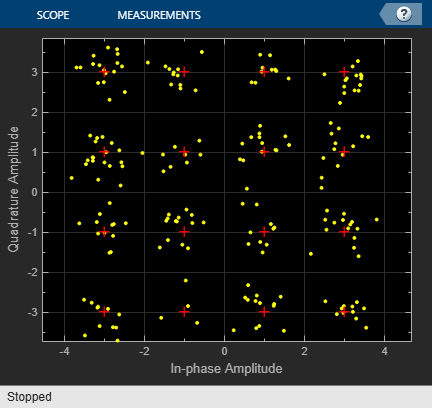# Rectangular QAM Modulator Baseband

Modulate using rectangular quadrature amplitude modulation

•Libraries:
Communications Toolbox / Modulation / Digital Baseband Modulation / AM
Communications Toolbox HDL Support / Modulation / AM

## Description

The Rectangular QAM Modulator Baseband block modulates the input signal using M-ary quadrature amplitude modulation with a constellation on a rectangular lattice. The output is a baseband representation of the modulated signal.

Note

All values of power assume a nominal impedance of 1 ohm.

## Examples

expand all

The `doc_qam_mod` model uses the Rectangular QAM Modulator Baseband block to modulate random data and applies noise to the signal by using the AWGN Channel block. After passing the symbols through a noisy channel, the model produces a constellation diagram of the noisy data by using the Constellation Diagram block. When you increase the noise level, the constellation points show increased signal distortion.

A Random Integer Generator block generates integers in the range [0,15] for a modulator configured to apply 16-QAM. The modulated signal passes through an AWGN channel, and a constellation diagram displays the resulting symbols.Run the model with Eb/N0 set to 20 dB in the AWGN channel.Change the Eb/No from 20 dB to 10 dB. Observe the increase in the noise.## Ports

### Input

expand all

Input signal, specified as a scalar or column vector. The input data must be binary values or integers in the range [0, (M – 1)], where M is the modulation order as specified by M-ary number.

Data Types: `single` | `double` | `int8` | `int16` | `int32` | `uint8` | `uint16` | `uint32` | `Boolean`

### Output

expand all

Rectangular QAM-modulated baseband signal, returned as a scalar or vector. Use the Output data type parameter to specify the output data type.

Data Types: `single` | `double` | `fixed point`

## Parameters

expand all

To edit block parameters interactively, use the Property Inspector. From the Simulink® Toolstrip, on the Simulation tab, in the Prepare gallery, select .

### Main

Modulation order, specified as a positive integer power of two. The modulation order specifies the number of points in the signal constellation. For more information, see Constellation Size and Scaling.

Input type, specified as `Integer` or `Bit` to indicate whether the input consists of integers or groups of bits.

• To use `Integer`, the input signal must consist of integers in the range [0, (M – 1)]. M is M-ary number.

• To use `Bit`, the input signal must contain binary values, and the number of rows must be an integer multiple of log2(M).

Mapping order of the symbols, specified as `Gray`, `Binary`, or `User-defined`. This parameter determines how the block maps each symbol to a group of output bits or integer.

Constellation mapping that you define, specified as an M-element containing unique integer values in the range [0, (M – 1)]. M is M-ary number.

The first element of this vector corresponds to the top-leftmost point of the constellation, with subsequent elements running down column-wise, from left to right. The last element corresponds to the bottom-rightmost point.

#### Dependencies

This parameter appears when you set Constellation ordering to `User-defined`.

Scaling method for constellation, specified as ```Min. distance between symbols```, ```Average Power```, or `Peak Power`. For more information, see Constellation Size and Scaling

Distance between the two nearest constellation points, specified as a positive scalar.

#### Dependencies

This parameter appears when you set Normalization method to ```Min. distance between symbols```.

Average power of the symbols in the constellation in watts, specified as a positive scalar. Power values assume a nominal impedance of 1 ohm.

#### Dependencies

This parameter appears when you set Normalization method to ```Average Power```.

Maximum power of the symbols in the constellation in watts, specified as a positive scalar. Power values assume a nominal impedance of 1 ohm.

#### Dependencies

This parameter appears when you set Normalization method to ```Peak Power```.

Rotation of the signal constellation in radians, specified as a numeric scalar.

Click on the block mask to visualize a signal constellation for the specified block parameters. Before viewing a constellation, apply the parameter settings. For more information, see View Constellation of Modulator Block.

### Data Types

Output data type, specified as `double`, `single`, `fixdt(1,16)`, `fixdt(1,16,0)`, ```Inherit: Inherit via back propagation```, or ```<data type expression>```.

• `fixdt(1,16)`, `fixdt(1,16,0)`, or `<data type expression>` enables parameters in which you can further specify details.

• ```Inherit: Inherit via back propagation``` sets the output data type and scaling to match the following block.

The Data Type Assistant helps you set data attributes. To use the Data Type Assistant, click. For more information, see Specify Data Types Using Data Type Assistant (Simulink).

## Block Characteristics

 Data Types `Boolean` | `double` | `fixed pointa, b, c` | `integer` | `single` Multidimensional Signals `no` Variable-Size Signals `yes` a Square QAM only.b ufix(1) at the input if ''input type'' is set to ''bit''. ufix(ceil(log2(M))) at input if ''input type'' is set to ''integer'' for M-ary modulation.c Fixed-point outputs must be signed.

## Algorithms

expand all

 Smith, J. G., "Odd-Bit Quadrature Amplitude-Shift Keying," IEEE Transactions on Communications, Vol. COM-23, March 1975, pp. 385–389.

 Proakis, John G. Digital Communications. 4th ed. New York: McGraw Hill, 2001.